# feature: an R package for feature significance for multivariate kernel density estimation

## Introduction

Feature significance is an extension of kernel density estimation which is used to establish the statistical significance of features (e.g. local modes). See Chaudhuri and Marronn (1999) for 1-dimensional data, Godtliebsen et al. (2002) for 2-dimensional data and Duong et al. (2007) for 3- and 4-dimensional data. The feature package contains a range of options to display and compute kernel density estimates, significant gradient and significant curvature regions. Significant gradient and/or curvature regions often correspond to significant features. In this vignette we focus on 1-, 2- and 3-dimensional data.

## Univariate data example

The earthquake data set contains 510 observations, each consisting of measurements of an earthquake beneath the Mt St Helens volcano. The first is the longitude (in degrees, where a negative number indicates west of the International Date Line), second is the latitude (in degrees, where a positive number indicates north of the Equator) and the third is the depth (in km, where a negative number indicates below the Earth’s surface). For the univariate example, we take the log(-depth) as our variable of interest. The kernel density estimate with bandwidth 0.1 is the orange curve. Superimposed in green are the sections of this density estimate which have significant gradient (i.e. significantly different from zero). The rug plot is the log(-depth) measurements.

library(feature)
data(earthquake)
eq3 <- log10(-earthquake[,3])
eq3.fs <- featureSignif(eq3, bw=0.1)
plot(eq3.fs, xlab="-log(-depth)", addSignifGradRegion=TRUE, addData=TRUE)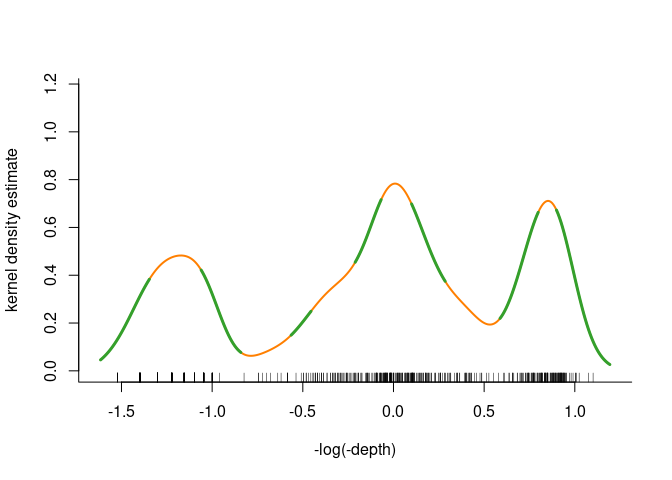xlim <- par()\$usr[1:2]  ## save x-axis limits to align following SiZer plot

Below this is the SiZer plot of Chaudhuri & Marron (1999). In the SiZer plot, blue indicates significantly increasing gradient, red is significantly decreasing gradient, purple is non-significant gradient and grey is data too sparse for reliable estimation. The horizontal black line is for the bandwidth 0.1.

eq3.SiZer <- SiZer(eq3, xlim=xlim, bw=c(0.05, 0.5), xlab="-log(-depth)")
abline(h=log(0.1))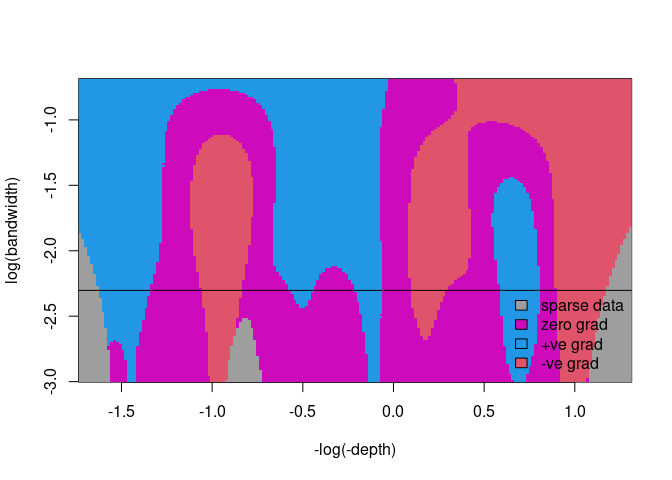## Bivariate data example

For bivariate data, we look at an Old Faithful geyser data set, in the MASS library. The horizontal axis is the waiting time (in minutes) between two eruptions, and the vertical axis is the duration time (in minutes) of an eruption. Below is a kernel density estimate with bandwidth (4.5, 0.37) with the significant curvature regions in blue superimposed.

library(MASS)
data(geyser)
geyser.fs <- featureSignif(geyser, bw=c(4.5, 0.37))
plot(geyser.fs, addSignifCurvRegion=TRUE)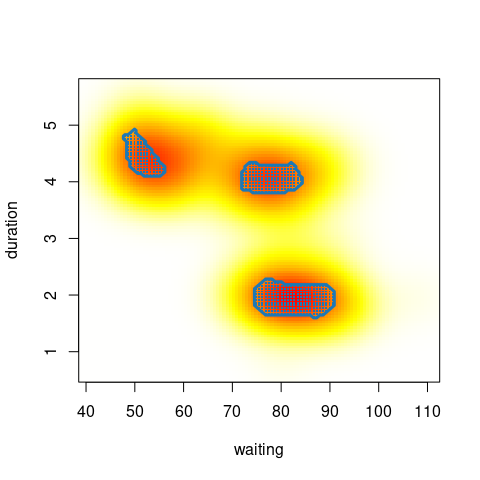A variation on plotting the significant regions is to plot the data points which fall inside these regions: significant curvature data points are in blue.

plot(geyser.fs, addSignifCurvData=TRUE)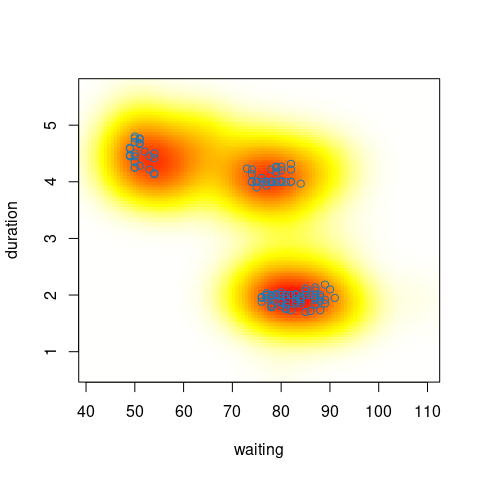## Trivariate data example

For trivariate data, we return to the earthquake data set. Below are the significant curvature regions in blue with bandwidth (0.06, 0.06, 0.05).

data(earthquake)
earthquake[,3] <- -log10(-earthquake[,3])
earthquake.fs <- featureSignif(earthquake, scaleData=TRUE, bw=c(0.06, 0.06, 0.05))
plot(earthquake.fs, addKDE=FALSE, addSignifCurvRegion=TRUE)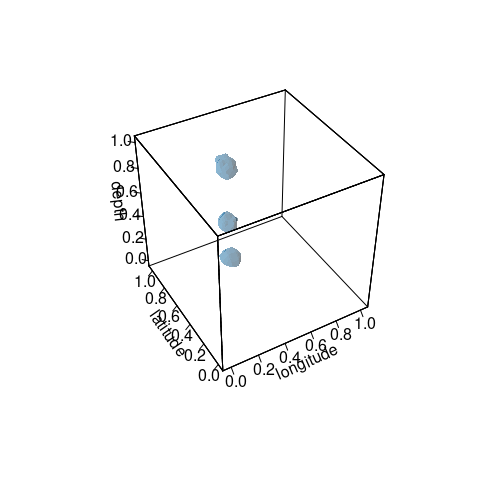The result of featureSignif is an object of class fs which is a list with fields

names(earthquake.fs)
#>   "x"              "names"          "bw"             "fhat"
#>   "grad"           "curv"           "gradData"       "gradDataPoints"
#>   "curvData"       "curvDataPoints"

where

• x is the data
• names are the name labels used for plotting
• bw is the bandwidth
• fhat is the kernel density estimate
• grad is the logical matrix indicating signficant gradient on a grid
• curv is the logical matrix indicating signficant curvature on a grid
• gradData is the logical vector indicating signficant gradient data points
• gradDataPoints are the signficant gradient data points
• curvData is the logical vector indicating signficant curvature data points
• curvDataPoints are the signficant curvature data points.

## Functionality not documented in this vignette

The function featureSignifGUI provides interactive feature significance via tcltk windows but the latter are not integrated with rmarkdown. See ?featureSignifGUI.